NEET  >  31 Year NEET Previous Year Questions: Waves - 1

# 31 Year NEET Previous Year Questions: Waves - 1

Test Description

## 11 Questions MCQ Test Physics Class 11 | 31 Year NEET Previous Year Questions: Waves - 1

31 Year NEET Previous Year Questions: Waves - 1 for NEET 2023 is part of Physics Class 11 preparation. The 31 Year NEET Previous Year Questions: Waves - 1 questions and answers have been prepared according to the NEET exam syllabus.The 31 Year NEET Previous Year Questions: Waves - 1 MCQs are made for NEET 2023 Exam. Find important definitions, questions, notes, meanings, examples, exercises, MCQs and online tests for 31 Year NEET Previous Year Questions: Waves - 1 below.
Solutions of 31 Year NEET Previous Year Questions: Waves - 1 questions in English are available as part of our Physics Class 11 for NEET & 31 Year NEET Previous Year Questions: Waves - 1 solutions in Hindi for Physics Class 11 course. Download more important topics, notes, lectures and mock test series for NEET Exam by signing up for free. Attempt 31 Year NEET Previous Year Questions: Waves - 1 | 11 questions in 20 minutes | Mock test for NEET preparation | Free important questions MCQ to study Physics Class 11 for NEET Exam | Download free PDF with solutions
 1 Crore+ students have signed up on EduRev. Have you?
31 Year NEET Previous Year Questions: Waves - 1 - Question 1

### Two waves are represented by the equations y1 = a sin (ωt + kx + 0.57) m and y2 = a cos (ωt + kx) m, where x is in meter and t in sec. The phase difference between them is 

Detailed Solution for 31 Year NEET Previous Year Questions: Waves - 1 - Question 1

Here, y1 = a sin (ωt + kx + 0.57)

and    y2 = a cos (ωt + kx)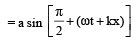Phase difference,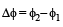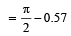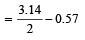= 1.57 – 0.57

31 Year NEET Previous Year Questions: Waves - 1 - Question 2

### Sound waves travel at 350 m/s through a warm air and at 3500 m/s through brass. The wavelength of a 700 Hz acoustic wave as it enters brass from warm air 

Detailed Solution for 31 Year NEET Previous Year Questions: Waves - 1 - Question 2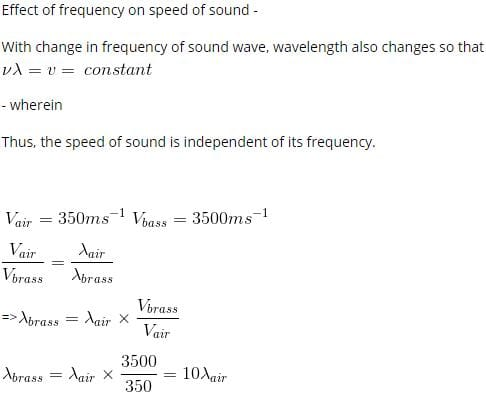31 Year NEET Previous Year Questions: Waves - 1 - Question 3

### Two identical piano wires kept under the same tension T have a fundamental frequency of 600 Hz. The fractional increase in the tension of one of the wires which will lead to occurrence of 6 beats/s when both the wires oscillate together would be [2011M]

Detailed Solution for 31 Year NEET Previous Year Questions: Waves - 1 - Question 3

For fundamental mode,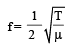Taking logarithm on both sides, we get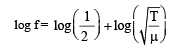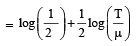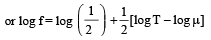Differentiating both sides, we get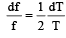(as  and μ are constants)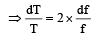Here df = 6

f = 600 Hz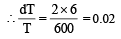31 Year NEET Previous Year Questions: Waves - 1 - Question 4

When a string is divided into three segments of length l1, l2, and l3  the fundamental frequencies of these three segments are v1, v2 and v3 respectively. The original fundamental frequency (v) of the string is 

Detailed Solution for 31 Year NEET Previous Year Questions: Waves - 1 - Question 4

Solution :- Let l be the length of the string

Frequency fundamental is given by (v) = 1/2l(T/u)^½

V is inversely proportional to the l

Here, l1 = k/v1 ; l2 = k/v2; l3 = k/v3; l =k/v

But l = l1 + l2 + l3

1/v = 1/v1 + 1/v2 + 1/v3

31 Year NEET Previous Year Questions: Waves - 1 - Question 5

Two sources of sound placed close to each other are emitting progressive waves given by y1 = 4 sin 600πt and y2 = 5 sin 608πt. An observer located near these two sources of sound will hear :

Detailed Solution for 31 Year NEET Previous Year Questions: Waves - 1 - Question 5

2πf1 = 600π
f1 = 300 ... (1)
2πf2 = 608π
f2 = 304 ...(2)
|f1 – f2| = 4 beats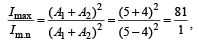where A1, A2 are amplitudes of given two sound wave.

31 Year NEET Previous Year Questions: Waves - 1 - Question 6

A train moving at a speed of 220 ms–1 towards a stationary object, emits a sound of frequency 1000 Hz. Some of the sound reaching the object gets reflected back to the train as echo. The frequency of the echo as detected by the driver of the train is : [2012M] (speed of sound in air is 330 ms–1)

Detailed Solution for 31 Year NEET Previous Year Questions: Waves - 1 - Question 6

Frequency of the echo detected by the driver of the train is (According to Doppler effect in sound)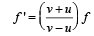where f = original frequency of source of sound f' = Apparent frequency of source because of the relative motion between source and observer.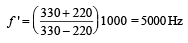31 Year NEET Previous Year Questions: Waves - 1 - Question 7

If we study the vibration of a pipe open at both ends, then which of the following statements is not true ? [NEET 2013]

Detailed Solution for 31 Year NEET Previous Year Questions: Waves - 1 - Question 7

Pressure change will be minimum at both ends. In fact, pressure variation is maximum at /2 because the displacement node is pressure antinode.

31 Year NEET Previous Year Questions: Waves - 1 - Question 8

A source of unknown frequency gives 4 beats/s, when sounded with a source of known frequency 250 Hz. The second harmonic of the source of unknown frequency gives five beats per second, when sounded with a source of frequency 513 Hz. The unknown frequency is [NEET 2013]

Detailed Solution for 31 Year NEET Previous Year Questions: Waves - 1 - Question 8

When sounded with a source of known frequency fundamental frequency = 250 ± 4 Hz = 254 Hz or 246 Hz
2nd harmonic if unknown frequency (suppose) 254 Hz = 2 × 254 = 508 Hz
As it gives 5 beats
∴  508 + 5 = 513 Hz
Hence, unknown frequency is 254 Hz

31 Year NEET Previous Year Questions: Waves - 1 - Question 9

A wave travelling in the +ve x-direction having displacement along y-direction as 1m, wavelen gth 2πm and frequency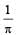Hz isrepresented by [NEET 2013]

Detailed Solution for 31 Year NEET Previous Year Questions: Waves - 1 - Question 9

As Y = A sin (ωt – kx + φ)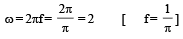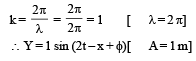31 Year NEET Previous Year Questions: Waves - 1 - Question 10

The length of the wire between two ends of a sonometer is 100 cm. What should be the positions of two bridges below the wire so that the three segments of the wire have their fundamental frequencies in the ratio of 1 : 3 : 5?              [NEET Kar. 2013]

Detailed Solution for 31 Year NEET Previous Year Questions: Waves - 1 - Question 10

Solution :- Fundamental frequency (v) = 1/L

As the fundamental frequencies are in the ratio of 1:3:5,

L1 : L2 : L3 = 1/1 : 1/3 : 1/5  => 15 : 5 : 3

Let x be the common factor , then

15x + 5x + 3x = L = 100

23x = 100

=> x = 100/23

L1 = 15*100/23 = 1500/23

L2 = 5*100/23 = 500/23

L3 = 3*100/23 = 300/23

The bridge should be placed from A at (1500/23 , 2000/23)

31 Year NEET Previous Year Questions: Waves - 1 - Question 11

Two sources P and Q produce notes of frequency 660 Hz each. A listener moves from P to Q with a speed of 1 ms–1. If the speed of sound is 330 m/s, then the number of beats heard by the listener per second will be [NEET Kar. 2013]

Detailed Solution for 31 Year NEET Previous Year Questions: Waves - 1 - Question 11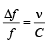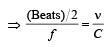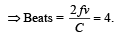## Physics Class 11

127 videos|464 docs|210 tests
 Use Code STAYHOME200 and get INR 200 additional OFF Use Coupon Code
Information about 31 Year NEET Previous Year Questions: Waves - 1 Page
In this test you can find the Exam questions for 31 Year NEET Previous Year Questions: Waves - 1 solved & explained in the simplest way possible. Besides giving Questions and answers for 31 Year NEET Previous Year Questions: Waves - 1, EduRev gives you an ample number of Online tests for practice

## Physics Class 11

127 videos|464 docs|210 tests

### How to Prepare for NEET

Read our guide to prepare for NEET which is created by Toppers & the best Teachers